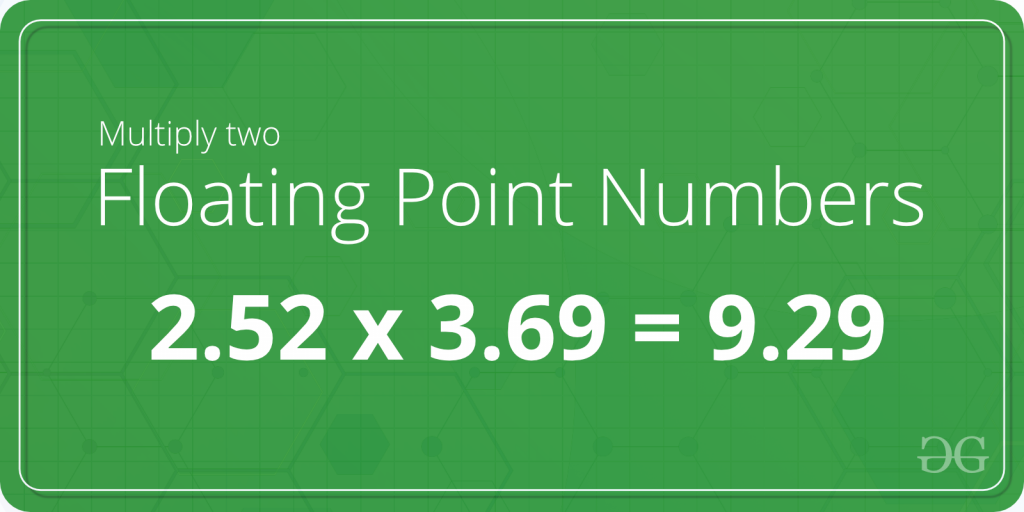# C Program to Multiply two Floating Point Numbers

• Difficulty Level : Basic
• Last Updated : 22 Apr, 2022

Given two floating numbers A and B. The task is to write a program to find the product of these two numbers.Examples:

Input: A = 2.12, B = 3.88 Output: 8.225600 Input: A = 3.78, B = 6.32 Output: 23.889601

In the below program to multiply two floating point numbers, the user is first asked to enter two floating numbers and the input is scanned using the scanf() function and stored in the variablesand. Then, the variablesandare multiplied using the arithmetic operatorand the product is stored in the variable product. Below is the C program to multiply two floating point numbers:

## C

 `// C program to multiply two floating point numbers``#include ` `int` `main()``{``    ``float` `A, B, product = 0.0f;` `    ``// Ask user to enter the two numbers``    ``printf``(``"Enter two floating numbers A and B : \n"``);` `    ``// Read two numbers from the user || A = 2.12, B = 3.88``    ``scanf``(``"%f%f"``, &A, &B);` `    ``// Calculate the multiplication of A and B``    ``// using '*' operator``    ``product = A * B;` `    ``// Print the product``    ``printf``(``"Product of A and B is: %f"``, product);` `    ``return` `0;``}`

Time Complexity: O(1), as we are not using any loops.

Auxiliary Space: O(1), as we are not using any extra space.

My Personal Notes arrow_drop_up